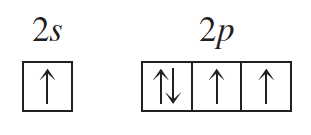# Problem: Given the valence electron orbital level diagram and the description, identify the atom in an excited state (assume two electrons occupy the 1s orbital):

⚠️Our tutors found the solution shown to be helpful for the problem you're searching for. We don't have the exact solution yet.

###### Problem Details

Given the valence electron orbital level diagram and the description, identify the atom in an excited state (assume two electrons occupy the 1s orbital):